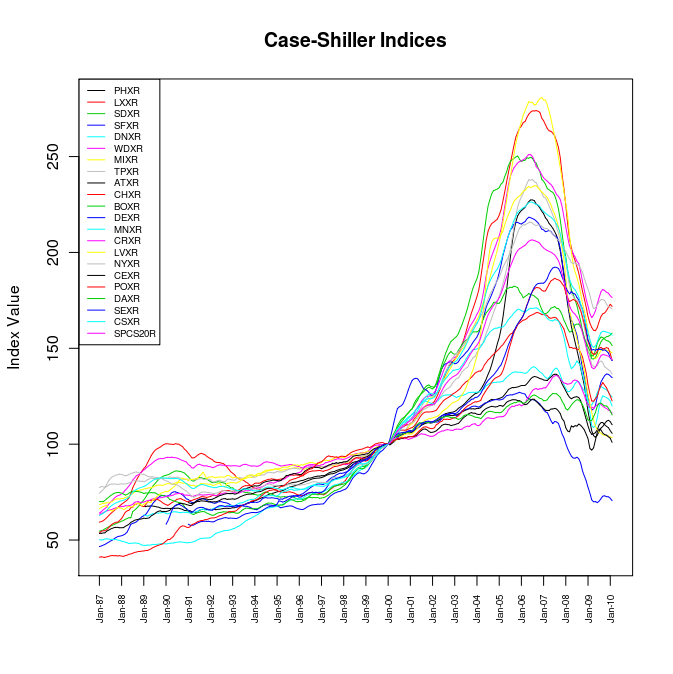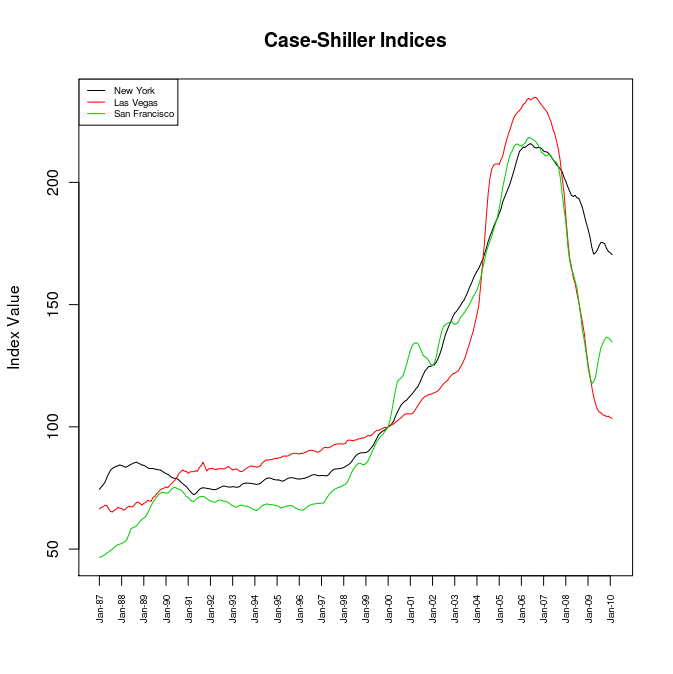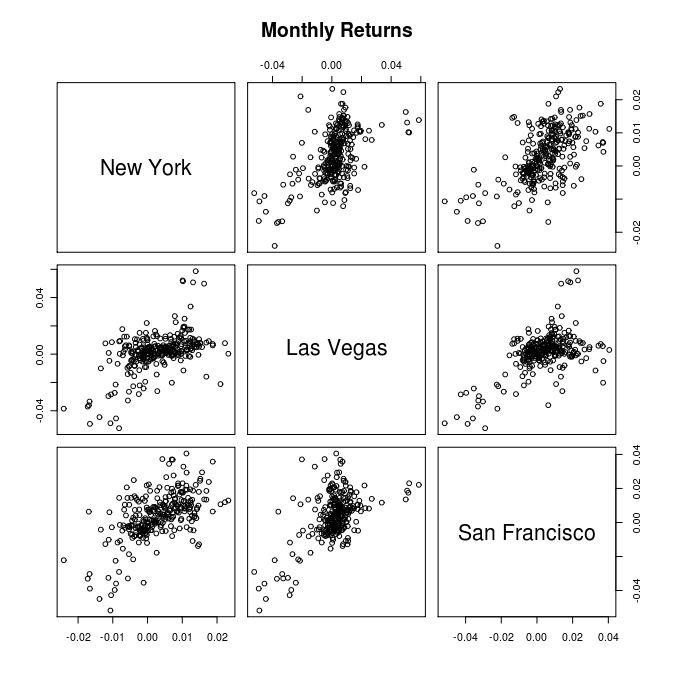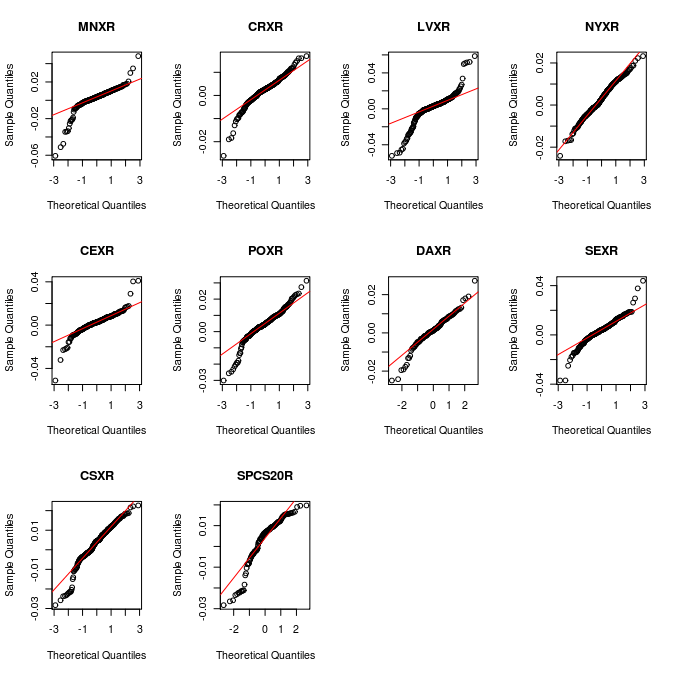Want to share your content on R-bloggers? click here if you have a blog, or here if you don't.

The Case-Shiller Home Price Indices measure residential home values for 20 cities in the US, with some indices going all the way back to the 80s. With housing prices all the rage these days, we should perform a quick-and-dirty analysis using R to see what we can glean from this rich dataset. First things first, the data needs to be downloaded from S&P’s website, converted into a CSV format, and then imported into R.

```## read in data dat <- read.csv("CSHomePrice_History.csv")```

Now that the data is loaded, lets start by simply plotting the time series of the Indices.

```## save dataset dimensions n <- dim(dat) m <- dim(dat)   ## plot time series col <- seq(1, m-1, 1) matplot(dat[,2:m], type="l", xaxt="n", main="Case-Shiller Indices", ylab="Index Value", lty=1, col=col) xticks <- seq(1, n, 12) xlabels <- dat\$YEAR[xticks] axis(1, at = xticks, las = 2, cex.axis = 0.6, labels = xlabels) legend("topleft", names(dat)[2:m], lty=1, cex=0.6, col=col)```There’s alot of ’stuff’ going on which makes it hard to distinguish one index from another. To simplify things, lets just plot a subset of the indices. For no particular reason, I’ll pick New York, Las Vegas, and San Francisco.

```## plot NY, LV, SF col <- seq(1, 3, 1) matplot(cbind(dat\$NYXR, dat\$LVXR, dat\$SFXR), type="l", xaxt="n", main="Case-Shiller Indices", ylab="Index Value", lty=1, col=col) xticks <- seq(1, n, 12) xlabels <- dat\$YEAR[xticks] axis(1, at = xticks, las = 2, cex.axis = 0.6, labels = xlabels) legend("topleft", c("New York", "Las Vegas", "San Francisco"), lty=1, cex=0.6, col=col)```Much better, but all this really shows us is that there was a pretty substantial run-up in home values starting in the late 90s, followed by a bust in 2006 (not exactly new news). What would be more interesting would be to analyze the monthly returns in the indices, which I suspect would be somewhat stationary. If we define$r_t$ as the monthly return in the form$x_{t+1} = x_t e^{r_t}$, we can calculate it as$r_t=ln (frac{x_{t+1}}{x_t})$. At this point we haven’t made any assumption about the distribution of$r_t$.

```## calculate the monthly returns r <- log(dat[2:n, 2:m] / dat[1:(n-1), 2:m])   ## plot monthly returns time series col <- seq(1, 3, 1) matplot(cbind(r\$NYXR, r\$LVXR, r\$SFXR), type="b", pch=21, xaxt="n", main="Monthly Returns", ylab="Monthly Return", lty=1, col=col) abline(h=0) xticks <- seq(2, n, 12) xlabels <- dat\$YEAR[xticks] axis(1, at = xticks, las = 2, cex.axis = 0.6, labels = xlabels) legend("bottomleft", c("New York", "Las Vegas", "San Francisco"), lty=1, cex=0.6, col=col)```Now things are starting to get interesting. Clearly there is some seasonality going on and the returns appear to be correlated. To investigate the correlation a bit more, lets do a pairs plot.

```## pairs plot of monthly returns pairs(cbind(r\$NYXR, r\$LVXR, r\$SFXR), main="Monthly Returns", labels=c("New York", "Las Vegas", "San Francisco"))```This confirms our suspicions about correlation. The monthly return almost appear bivariate normal. Lets produce some boxplots to investigate the distribution of$r_t$.

```## boxplot boxplot(r, xaxt="n", main="Monthly Returns", ylab="Monthly Return", col="light blue") abline(h=0) xticks <- seq(1, m-1, 1) xlabels <- names(r) axis(1, at = xticks, las = 2, cex.axis = 0.6, labels = xlabels)```It appears that the returns are roughly normal, with the mean return just above 0, but some appear to have much fatter tails than others (compare New York to Las Vegas for instance). We should perform some QQ Normal plots to see how normal the monthly returns really are.

```## qqnorm plots par(mfrow=c(3,4)) for(i in 1:12){ qqnorm(r[,i], main=names(r)[i]) qqline(r[,i], col="red") } windows() par(mfrow=c(3,4)) for(i in 13:(m-1)){ qqnorm(r[,i], main=names(r)[i]) qqline(r[,i], col="red") }```This confirms our suspicions that the return are ‘normal like’, but have some pretty fat tails (as do most financial assets). Although, New York and Boston appear to be much more normal than the rest. This analysis really begs for an ARMA model that incorporates the correlation across housing markets.